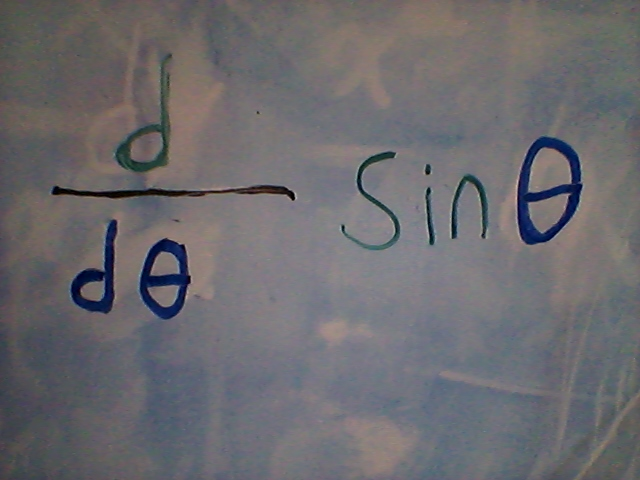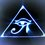# Derivative of The Sine FunctionIn my last note I proved that $\quad\displaystyle \lim_{\theta\to 0}\frac { \theta}{sin\theta} = 1$

If you have not read that I would recommend reading that first as this limit is used in proving the derivative of the sine function. Here is the link.

$\qquad \qquad\qquad\qquad\qquad\qquad \displaystyle \lim_{\theta\to 0}\frac { \theta}{sin\theta} = ?$

To prove the derivative of the sine function we will be using the first principles of derivatives.

$\qquad\qquad\qquad\qquad\qquad\displaystyle \lim_{\Delta\theta\to 0}\frac{\sin(\theta + \Delta\theta) - \sin\theta}{\Delta\theta}$

Use the addition formula for $\sin(\theta + \Delta\theta)$.

$=\qquad\qquad\qquad\quad\displaystyle\lim_{\Delta\theta\to 0} \frac{\sin\theta \times \cos \Delta\theta + \sin\Delta\theta \times \cos\theta - \sin\theta}{\Delta\theta}$

rearrange the equation and factor out $\sin\theta$

$=\qquad\qquad\qquad\quad\displaystyle\lim_{\Delta\theta\to 0} \frac{\sin\theta \times \cos \Delta\theta - \sin\theta + \sin\Delta\theta \times \cos\theta }{\Delta\theta}$

$=\qquad\qquad\qquad\quad\displaystyle\lim_{\Delta\theta\to 0} \frac{\sin\theta( \cos \Delta\theta - 1) + \sin\Delta\theta \times \cos\theta }{\Delta\theta}$

break apart the fraction

$=\qquad\qquad\qquad\quad\displaystyle \lim_{\Delta\theta\to 0}\frac{\sin\theta( \cos \Delta\theta - 1)}{\Delta\theta} + \frac{\sin\Delta\theta \times \cos\theta }{\Delta\theta}$

$=\qquad\qquad\qquad\quad\displaystyle \lim_{\Delta\theta\to 0}\sin\theta \times \frac{( \cos \Delta\theta - 1)}{\Delta\theta} + \frac{sin\Delta\theta}{\Delta\theta} \times cos\theta$

We wIll now evaluate the two limits seperately

$\qquad\qquad\qquad\quad\displaystyle \lim_{\Delta\theta\to 0} \frac{sin\Delta\theta}{\Delta\theta} \times cos\theta$

In my last note I proved that $\displaystyle\lim_{\Delta\theta\to 0}\frac{sin\Delta\theta}{\Delta\theta}=1$

$=\qquad\qquad\qquad\quad\displaystyle \lim_{\Delta\theta\to 0} 1 \times cos\theta$

$=\qquad\qquad\qquad\quad\displaystyle \lim_{\Delta\theta\to 0} cos\theta$

That solves that limit now the other limit.

$\qquad\qquad\qquad\quad\displaystyle \lim_{\Delta\theta\to 0}\sin\theta \times \frac{( \cos \Delta\theta - 1)}{\Delta\theta}$

divide out the $\sin\theta$

$=\qquad\qquad\qquad\quad\displaystyle \sin\theta \lim_{\Delta\theta\to 0} \frac{( \cos \Delta\theta - 1)}{\Delta\theta}$

multiply by the conjugate

$=\qquad\qquad\qquad\quad\displaystyle \sin\theta \lim_{\Delta\theta\to 0} \frac{( \cos \Delta\theta - 1)}{\Delta\theta} \times \frac{\cos\Delta\theta+1}{\cos\Delta\theta+1}$

$=\qquad\qquad\qquad\quad\displaystyle \sin\theta \lim_{\Delta\theta\to 0} \frac{( \cos^{2} \Delta\theta - 1)}{\Delta\theta(\cos\Delta\theta+1)}$

Rewrite the the equation using the Pythagorean identity. $\cos^{2}\theta + \sin^{2}\theta=1$

$=\qquad\qquad\qquad\quad\displaystyle \sin\theta \lim_{\Delta\theta\to 0} \frac{-\sin^{2} \Delta\theta }{\Delta\theta(\cos\Delta\theta+1)}$

break apart the fraction

$=\qquad\qquad\qquad\quad\displaystyle \sin\theta \lim_{\Delta\theta\to 0} (-sin\Delta\theta) \times \frac{\sin\Delta\theta}{\Delta\theta} \times \frac{1}{\cos\Delta\theta + 1}$

evaluate the limits

$=\qquad\qquad\qquad\quad\displaystyle \sin\theta \times (-sin0) \times 1 \times \frac{1}{\cos0 + 1}$

$=\qquad\qquad\qquad\quad\displaystyle \sin\theta \times 0 \times 1 \times \frac{1}{2}$

$=\qquad\qquad\qquad\quad\displaystyle 0$

$\displaystyle \frac{d}{d\theta}=\qquad\qquad\qquad\quad \lim_{\Delta\theta\to 0}\sin\theta \times \frac{( \cos \Delta\theta - 1)}{\Delta\theta} + \lim_{\Delta\theta\to 0}\frac{sin\Delta\theta}{\Delta\theta} \times cos\theta$

$\displaystyle \frac{d}{d\theta}=\qquad\qquad\qquad\quad0 + \cos\theta$

$\displaystyle \frac{d}{d\theta}=\qquad\qquad\qquad\quad \cos\theta$Note by Brody Acquilano
6 years, 2 months ago

This discussion board is a place to discuss our Daily Challenges and the math and science related to those challenges. Explanations are more than just a solution — they should explain the steps and thinking strategies that you used to obtain the solution. Comments should further the discussion of math and science.

When posting on Brilliant:

• Use the emojis to react to an explanation, whether you're congratulating a job well done , or just really confused .
• Ask specific questions about the challenge or the steps in somebody's explanation. Well-posed questions can add a lot to the discussion, but posting "I don't understand!" doesn't help anyone.
• Try to contribute something new to the discussion, whether it is an extension, generalization or other idea related to the challenge.

MarkdownAppears as
*italics* or _italics_ italics
**bold** or __bold__ bold
- bulleted- list
• bulleted
• list
1. numbered2. list
1. numbered
2. list
Note: you must add a full line of space before and after lists for them to show up correctly
paragraph 1paragraph 2

paragraph 1

paragraph 2

[example link](https://brilliant.org)example link
> This is a quote
This is a quote
    # I indented these lines
# 4 spaces, and now they show
# up as a code block.

print "hello world"
# I indented these lines
# 4 spaces, and now they show
# up as a code block.

print "hello world"
MathAppears as
Remember to wrap math in $$ ... $$ or $ ... $ to ensure proper formatting.
2 \times 3 $2 \times 3$
2^{34} $2^{34}$
a_{i-1} $a_{i-1}$
\frac{2}{3} $\frac{2}{3}$
\sqrt{2} $\sqrt{2}$
\sum_{i=1}^3 $\sum_{i=1}^3$
\sin \theta $\sin \theta$
\boxed{123} $\boxed{123}$